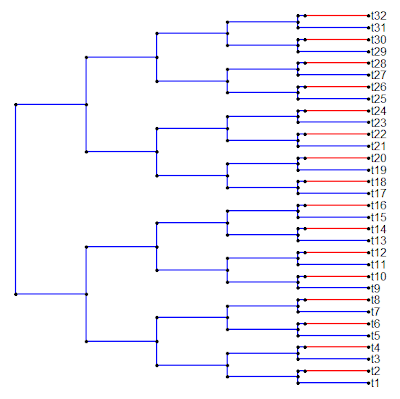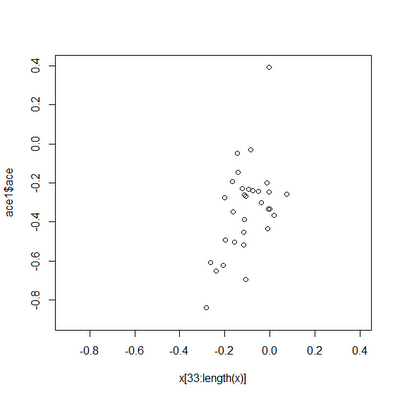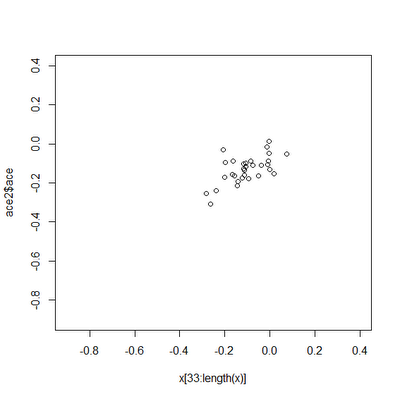## Wednesday, December 7, 2011

### Ancestral state estimation when the rate of evolution varies throughout the tree

A user contacting me recently about performing ancestral state estimation when the rate of evolution varies on the different branches of the tree. (Actually, she contacted me about another, slightly more complex problem - but this is where I'm starting.) To see why this might be an issue, consider the following tree:and imagine that the rate of evolution is very low [say σ(blue)2=0.01] on the blue branches of the tree, and very high [say σ(red)2=1.0] on the red branches. Under this scenario, the states at the internal nodes of the tree will be much closer in value to the state at the root node than they will be (on average) to any red tip. We might like to take this into consideration in estimating ancestral character values.

To show this, let's consider the following example. First, let's simulate data on the tree by first stretching the branches of the tree by their rates of evolution, then by using fastBM(). Here I use fastBM() rather than the much easier phytools function sim.rates(), because fastBM() allows us to return the states at internal nodes. Then, I will use anc.ML() to estimate internal node states using likelihood, ignoring heterogeneity in the evolutionary rate, and compare these estimates to the "true," normally unknown, ancestral character values.

> require(phytools)
> source("anc.ML.R")
> cols<-c("blue","red"); names(cols)<-c(1,2)
> sig2<-c(0.01,1); names(sig2)<-c(1,2)
> simtree<-tree; simtree\$edge.length<-colSums(t(tree\$mapped.edge)*sig2)
> x<-fastBM(simtree,internal=T) # parts of Newick style tree not shown
> ace1<-anc.ML(tree,x[1:32])
> mse1<-mean((x[33:length(x)]-ace1\$ace)^2)
> mse1
 0.09184913
> plot(x[33:length(x)],ace1\$ace)Obviously, these estimates are not great, and the ancestral state estimates (on the y-axis) span a much broader range than the true values, as we might expect. [I have plotted the points on a square set of axes just to make this point clear.] Now, for comparison, let's first fit a multi-rate BM model and then try to use the fitted rates to inform our ancestral character estimation on the tree:

> fit<-brownie.lite(tree,x[1:32])
> fit
...
\$sig2.multiple
1 2
0.005546321 0.684734146
...
\$P.chisq
 2.143502e-11
> fittree<-tree
> fittree\$edge.length<- colSums(t(tree\$mapped.edge)*fit\$sig2.multiple)
> ace2<-anc.ML(fittree,x[1:32])
> mse2<-mean((x[33:length(x)]-ace2\$ace)^2)
> mse2
 0.005524579
> plot(x[33:length(x)],ace2\$ace)Clearly, the estimates are much closer to the true values in this case.

To be perfectly transparent, this particular example is designed to be an extreme case, and for most trees & datasets, the effect will be much smaller.

#### 1 comment:

1.I don't have much to say other than that is awesome.

Note: due to the very large amount of spam, all comments are now automatically submitted for moderation.